# Calculate Percentage Increase in Excel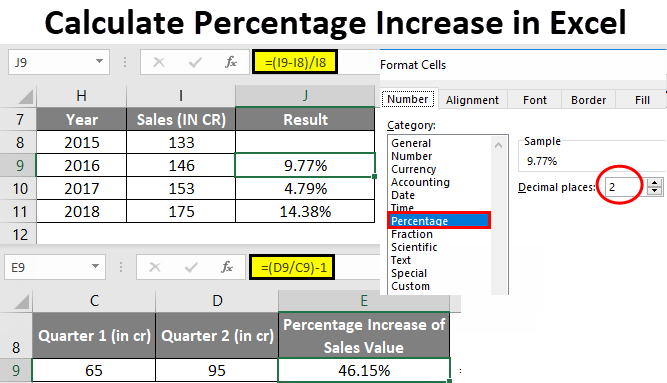• Calculate Percentage Increase in Excel
• How to Calculate a Percentage Increase in Excel?

## Calculate Percentage Increase in Excel

• Usually, in Excel, it doesn’t provide any inbuilt function to calculate percentage changes i.e. Percentage increase.
• Percentage change formula is most commonly & very often used in Excel across financial departments in the companies.
• The percentage increase is used to track the business results each month for an organization, which can be a month on month, quarter on quarter and yearly sales.
• Percentage increase calculation is most commonly used in sales reporting, where you will come to know the sales growth Quarter on Quarter or Year on Year.
• Percentage increase calculation is used in Sales Tax Calculation where you will add-in a sale tax to an invoice total or sales total.
• Prior to knowing about Percent increase or change formula, you should be aware of Percentage basics in excel.

Mathematical percentage calculation: (Part/Whole) *100 = Percentage

For example, suppose if you had 30 pens and you gave 6 pens to your best friends.

If anybody asks you, how much did you give by Percentage wise? It can be calculated by the above-mentioned formula.

By performing a simple calculation =6/30*100 you get the answer as 20%.

This is how we normally calculate percentages everywhere and in everyday life.

In Excel, the basic formula to calculate percentage is

Excel percentage calculation: Part/Total = Percentage

The only difference between Mathematical & excel percentage calculation is, In excel *100 is missing, because in Excel when calculating a percent, you don’t have to multiply the resulting value fraction with 100, since, excel will automatically calculate it to or convert it to percentage format, which will be applied to a cell.

Calculate a Percentage Increase in Excel

To increase value or to calculate the amount of percentage increase in excel, below mentioned formulas are used

• = Amount * (1 + %) or
• =(new_value/old_value)-1 or
• =(new value – old value)/old value

### How to Calculate a Percentage Increase in Excel?

To Calculate a percentage Increase in Excel is very simple and easy. Let’s check out various available options or formula on how to calculate a percentage increase in Excel.

### Example #1 – Calculate Percentage Increase Between any two numbers in Excel

In the below-mentioned example, I have a two quarterly sales values in the cell “C9” & “D9” i.e. (First quarter sales value in the cell C9 & Second quarter sales value in the cell D9)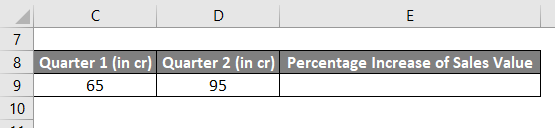Here I need to calculate the percentage increase in Second quarter sales compared to the First quarter sales value in excel.

A below-mentioned formula is used to calculate the percentage increase value between two numbers or values in excel.

=(new_value/old_value)-1

Select a blank cell where I need to enter the formula i.e. I selected the cell E9 where I will enter =(D9/C9)-1, where “new_value” is 95 or I can enter cell reference i.e. “D9” and “old_value” is 65 or I can enter cell reference i.e. “C9”. These are the two numbers or values which I need to compare.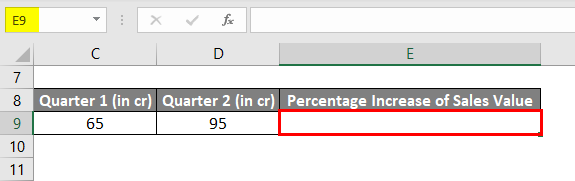With First quarter sales value in the cell “C9” & Second quarter sales value in the cell “D9”, I entered =(D9/C9)-1 in cell “E9” to find out the percentage increase in quarter 2.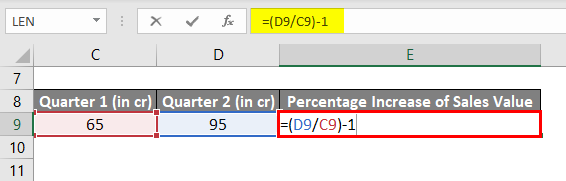It results in the value 0.46,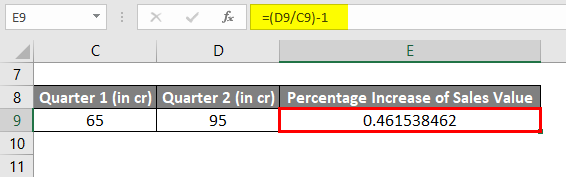I need to convert it to a percentage value. Below mentioned steps are used to convert the result value from decimal format to a percentage format:

I need to select the cell E9, Right-click on the cell E9 and I need to select Format Cells option to change the result from a decimal format to a percentage format.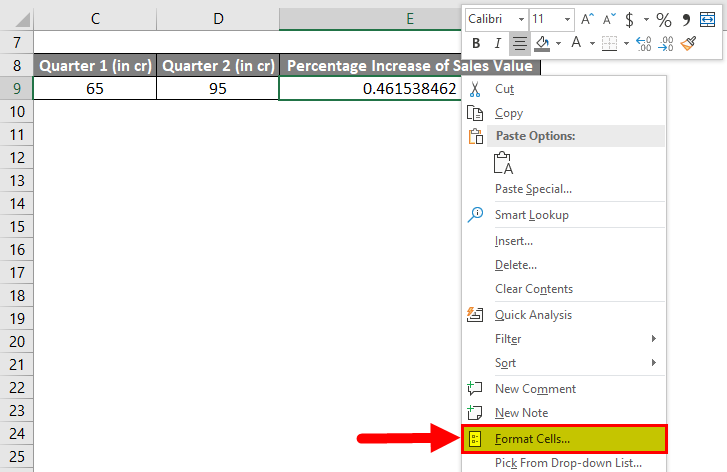Format Cells window appears, in the category section, I need to select Percentage, and decimal places, I need to select 2 by clicking on small arrow around decimal places, and click OK.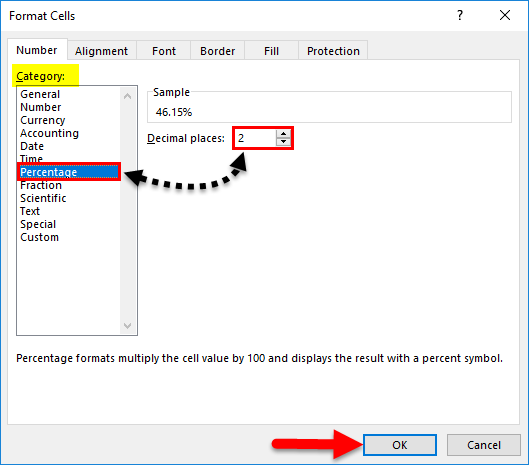Now, In the cell E9, you can check out where the decimal format gets converted to percentage format i.e. 0.4615 to 46.15%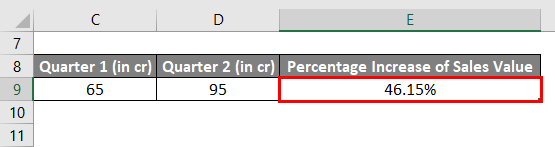Note: If quarterly sales value is decreased in quarter 2 or if the second quarter sales value is less than the first quarter sales value, then it will result in negative value or number which indicates a decline in sales.

### Example #2 – Calculate Percentage Increase Between yearly sales data in Excel

In the below-mentioned example, I have yearly sales data table where it contains two columns, i.e. Year & its sales values for the respective year.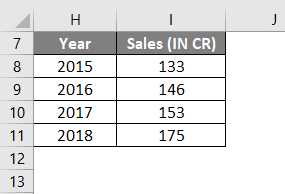Here, in comparison with the previous year, I need to calculate a percentage increase in sales value in excel. It can be done by below-mentioned formula.

=(new value–old value)/old value

I can start to find out the percentage increase in sales value from 2016 with reference to the year 2015, i.e. in cell J9, I entered the above-mentioned formula.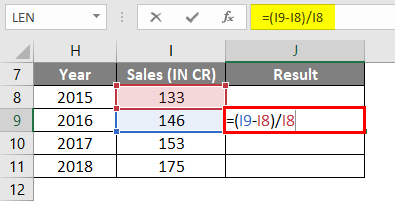Which result in 0.097.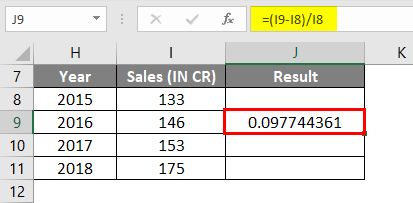Simultaneously, this formula is applied to the whole range, i.e. from the cell J9 to J11, by selecting the cell J9 and drag it to the J11.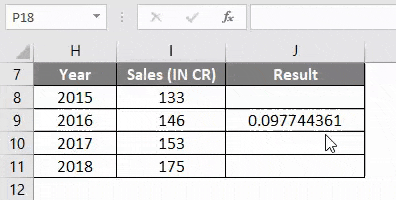Usually, the result of percentage change, appear in decimal format in excel. Later it is converted to percentage format, the same procedure needs to be followed which I have explained in the first example (Conversion of result value from decimal format to a percentage format)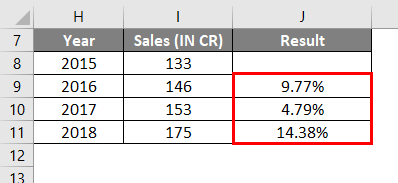### Example #3 – Calculate to increase a number by a specific percentage in Excel

To increase value or number by a specific percentage, below mentioned formula is used:

=Amount*(1+%)

In the below-mentioned example, I have a quarter 2 sales data in the cell C14, where I need to increase it by 20% value.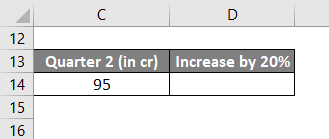The below-mentioned formula is used to get the desired output.

=C14*(1+20%)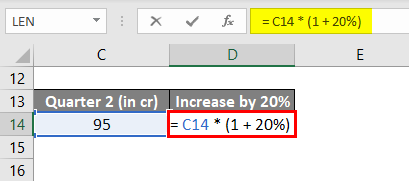It results in or increases the sales value in cell D14 by 20% i.e. 114.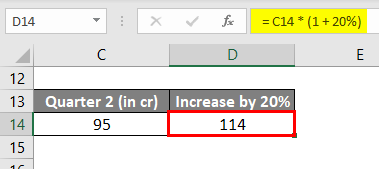### Things to Remember

• Decimal format to a percentage format can also be done with the help of shortcut key CTRL + SHIFT + % (Note: it applies the percentage format with no decimal places).
• Usually, Percentage value which occurs during end result in Excel are stored as decimal values or format, we have to convert it into percentage format.
• Decimal format to Percentage Format conversion can be done either through the Ribbon Options or through the Format Cells Dialog Box.
• #DIV/0! Error occurs if we try to divide by zero in percentage change excel formula =(b-a)/0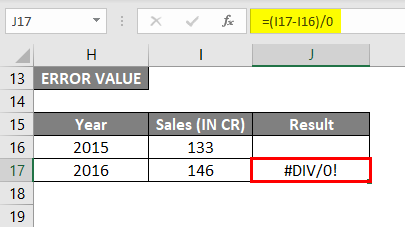You can download this Calculate Percentage Increase Excel Template here – Calculate Percentage Increase Excel Template

### Recommended Articles

This has been a guide to Calculate Percentage Increase in Excel. Here we discuss how to calculate a percentage increase in excel along with excel examples and downloadable excel template. You can also go through our other suggested articles –

1. Interest Rate vs Annual Percentage Rate
2. Learn Dynamic Tables in Excel
3. How to use Divide Formula in Excel
4. Subtraction in Excel | Easy Excel Tutorials

The post Calculate Percentage Increase in Excel appeared first on EDUCBA.

This post first appeared on Best Online Training & Video Courses | EduCBA, please read the originial post: here